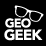# Convert polygon outlines into points

8243
13
12-26-2014 04:53 PMOccasional Contributor

Hi,

Is there anyway to convert polygon outlines into points, preferably with the ability to decide the gap of each point to be placed (just like the "construct points" from lines tool)?

The purpose is for creating service areas around polygons in Network Analyst. Or if there is another method of doing this besides the conversion do let me know!

I am using ArcGIS 10.2.2.

Thanks!

Tags (2)
13 RepliesOccasional Contributor II

Hi Gaston

i done it with QGIS:byEsri Esteemed Contributor

The da.SearchCursor could be easily replaced with the "old" SearchCursor, but what would be more work is to rebuild the positionAlongLine method, which is not available at 10.0. You may want to post your own question with that specific detail. I guess that would attract more people to help you with that problem. However, I will have a look to see if I can easily solve it, but I do not have access to 10.0 anymore.

Kind regards, XanderbyEsri Esteemed Contributor

An alternative would be to use the Densify tool to create points every x meter on the line followed by te Feature Vertices to Points tool (if you have access to those tolos).byEsri Esteemed Contributor

Ok, I just tried some code using (I think) only 10.0 functionality. So Gaston Izaguirre‌, if you want to try, give it a shot here is some code:

Change the code on line 3 and 4 to point to your input polyline featureclass and output point featureclass (output will be created, if it exists it raises an error). Line 5 contains the interval size (distance between each point) and line 6 indicates if the last point should be added too (True).

```import arcpy, math, numpy
def main():
fc_in = r"D:\Xander\GeoNet\Polyline2Points10.0\test.gdb\lines_diss_projected"
fc_out = r"D:\Xander\GeoNet\Polyline2Points10.0\test.gdb\lines_diss_toPnts01"
interval = 100

fld_shp = arcpy.Describe(fc_in).shapeFieldName
sr = arcpy.Describe(fc_in).spatialReference
curs = arcpy.SearchCursor(fc_in)
pnts = []
for row in curs:
polyline = row.getValue(fld_shp)
d = 0
frac_left = 0

for p in range(0, polyline.partCount):
part = polyline.getPart(p)
for i in range(0, len(part)-1):
pnt1 = part
pnt2 = part[i+1]
length_part = getDistance2Points(pnt1, pnt2)

for frac in numpy.arange(frac_left, length_part, interval):
pnt = getPointBasedOnFractionOfLine(pnt1, pnt2, frac)
pntg = arcpy.PointGeometry(pnt, sr)
pnts.append(pntg)
frac_left = interval - (length_part - frac)

pnts.append(arcpy.PointGeometry(polyline.lastPoint, sr))
del curs, row

# write list to output featureclass
arcpy.CopyFeatures_management(pnts, fc_out)

def getDistance2Points(pnt1, pnt2):
return math.hypot(pnt1.X-pnt2.X, pnt1.Y-pnt2.Y)

def getPointBasedOnFractionOfLine(pnt1, pnt2, frac):
length_line = getDistance2Points(pnt1, pnt2)
f = frac / length_line
return arcpy.Point(pnt2.X * f + pnt1.X * (1-f), pnt2.Y * f + pnt1.Y * (1-f))

if __name__ == '__main__':
main()```